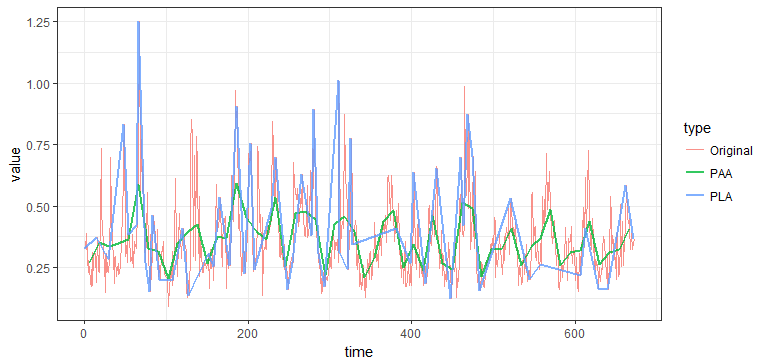# TSrepr - R package for time series representations

TSrepr is R package for fast time series representations and dimensionality reduction computations (i.e. time series feature extraction). Z-score normalisation, min-max normalisation, forecasting accuracy measures and other useful functions implemented in C++ (Rcpp) and R. TSrepr package is available on CRAN.

## Installation

You can install TSrepr directly from CRAN:

Or development version from GitHub with:

## Overview

All type of time series representations methods are implemented, and these are so far:

• PAA - Piecewise Aggregate Approximation (repr_paa)
• DWT - Discrete Wavelet Transform (repr_dwt)
• DFT - Discrete Fourier Transform (repr_dft)
• DCT - Discrete Cosine Transform (repr_dct)
• SMA - Simple Moving Average (repr_sma)
• PIP - Perceptually Important Points (repr_pip)
• SAX - Symbolic Aggregate Approximation (repr_sax)
• PLA - Piecewise Linear Approximation (repr_pla)
• Model-based:
• Mean seasonal profile - Average seasonal profile, Median seasonal profile, etc. (repr_seas_profile)
• Model-based seasonal representations based on linear (additive) model (LM, RLM, L1, GAM) (repr_lm, repr_gam)
• Exponential smoothing seasonal coefficients (repr_exp)
• Data dictated:
• FeaClip - Feature extraction from clipping representation (repr_feaclip, clipping)
• FeaTrend - Feature extraction from trending representation (repr_featrend, trending)
• FeaClipTrend - Feature extraction from clipping and trending representation (repr_feacliptrend)

Additional useful functions are implemented as:

• Windowing (repr_windowing) - applies above mentioned representations to every window of a time series
• Matrix of representations (repr_matrix) - applies above mentioned representations to every row of a matrix of time series
• Normalisation functions - z-score (norm_z), min-max (norm_min_max)
• Normalisation functions with output also of scaling parameters - z-score (norm_z_list), min-max (norm_min_max_list)
• Denormalisation functions - z-score (denorm_z), min-max (denorm_min_max)
• Forecasting accuracy measures - MSE, MAE, RMSE, MdAE, MAPE, sMAPE, MAAPE, MASE

## Usage